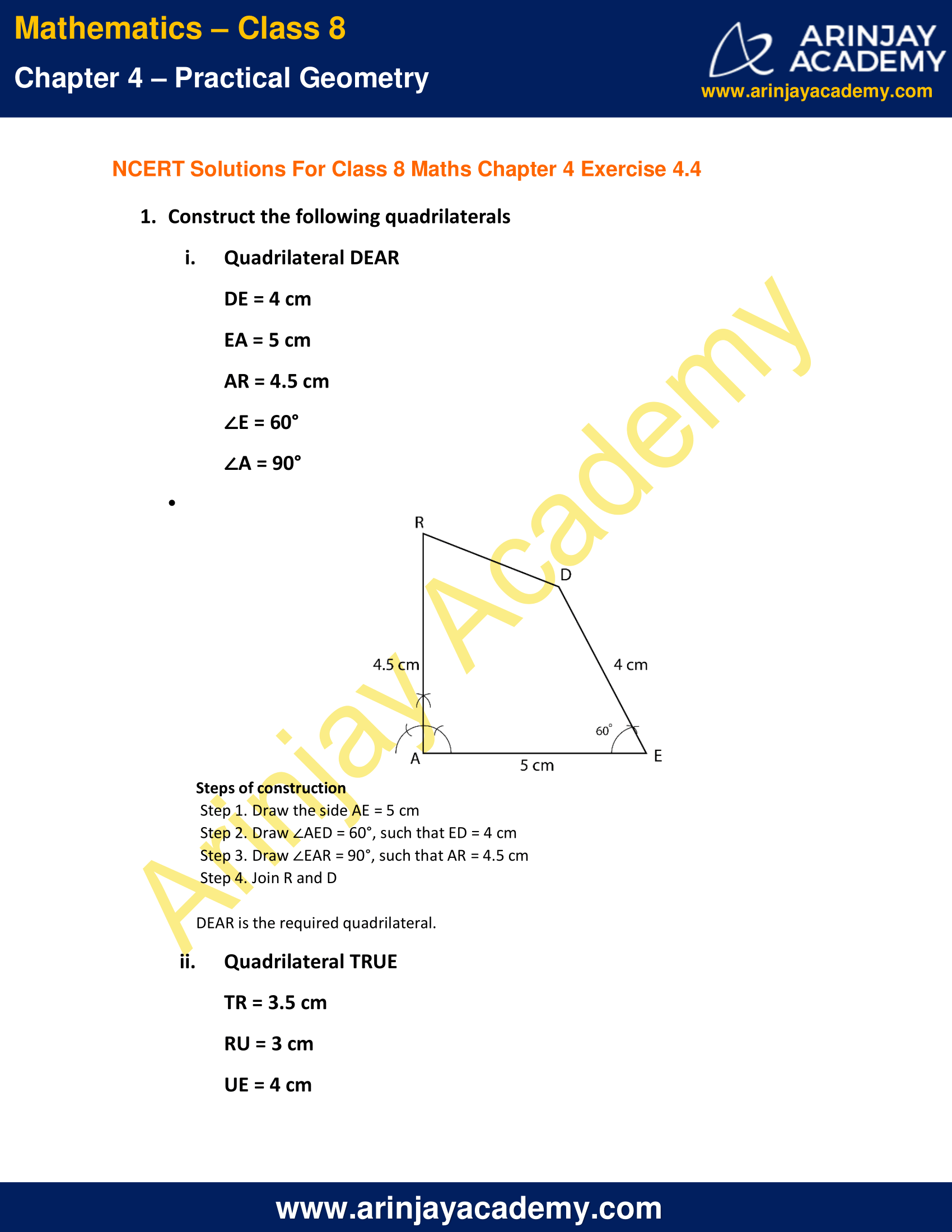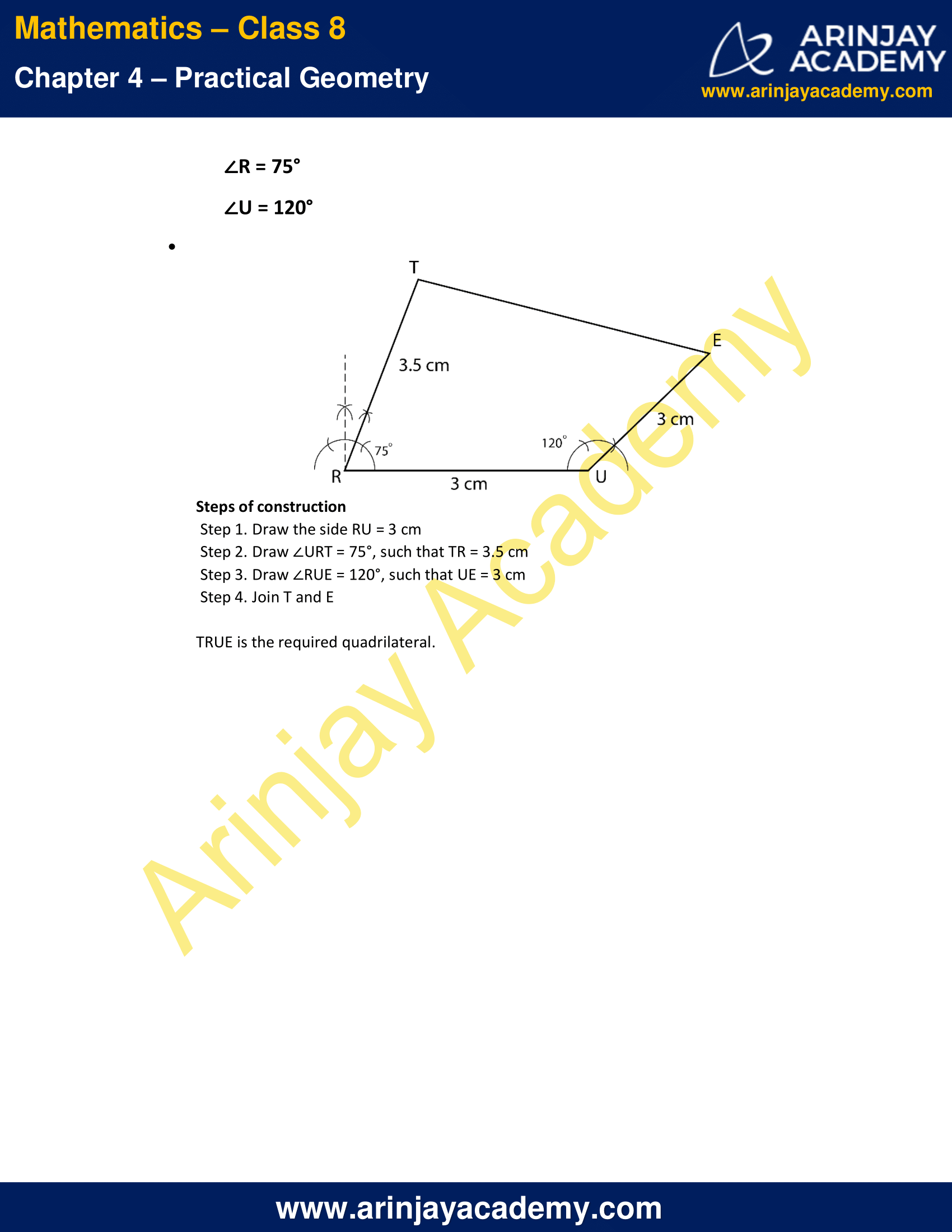# NCERT Solutions for Class 8 Maths Chapter 4 Exercise 4.4 – Practical Geometry

NCERT Solutions for Class 8 Maths Chapter 4 Exercise 4.4 – Practical Geometry, has been designed by the NCERT to test the knowledge of the student on the topic – Constructing a Quadrilateral – When three sides and two included angles are given

### NCERT Solutions for Class 8 Maths Chapter 4 Exercise 4.4 – Practical GeometryNCERT Solutions for Class 8 Maths Chapter 4 Exercise 4.4 – Practical Geometry

1. Construct the following quadrilaterals

i. Quadrilateral DEAR
DE = 4 cm
EA = 5 cm
AR = 4.5 cm
∠E = 60°
∠A = 90°

Solution:Steps of construction

• Step 1. Draw the side AE = 5 cm
• Step 2. Draw ∠AED = 60°, such that ED = 4 cm
• Step 3. Draw ∠EAR = 90°, such that AR = 4.5 cm
• Step 4. Join R and D

DEAR is the required quadrilateral.

ii. Quadrilateral TRUE

TR = 3.5 cm
RU = 3 cm
UE = 4 cm
∠R = 75°
∠U = 120°

Solution:Steps of construction

• Step 1. Draw the side RU = 3 cm
• Step 2. Draw ∠URT = 75°, such that TR = 3.5 cm
• Step 3. Draw ∠RUE = 120°, such that UE = 3 cm
• Step 4. Join T and E

TRUE is the required quadrilateral.

The next Exercise for NCERT Solutions for Class 8 Maths Chapter 4 Exercise 4.5 – Practical Geometry can be accessed by clicking here